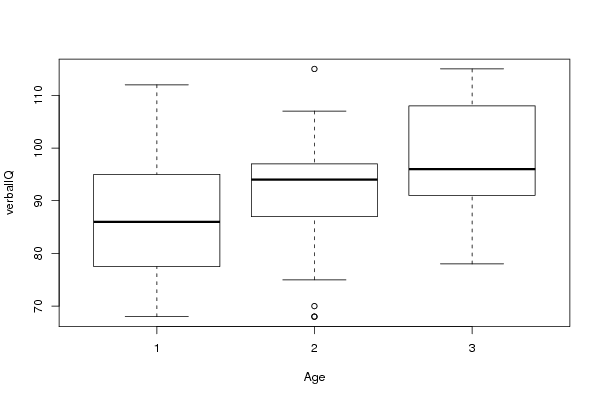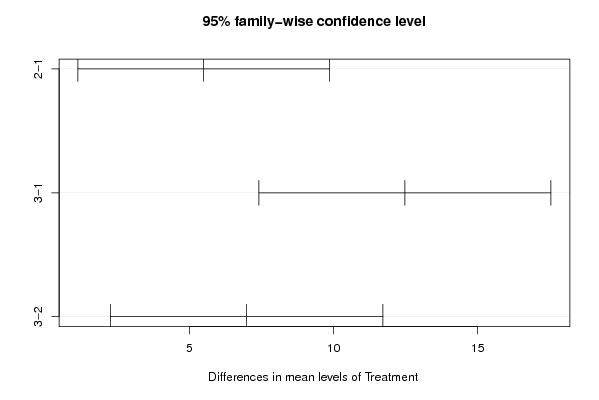## Free Statistics

of Irreproducible Research!

Author's title
Author*The author of this computation has been verified*
R Software ModuleIan.Hollidayrwasp_One Factor ANOVA.wasp
Title produced by softwareChi Square Measure of Association- Free Statistics Software (Calculator)
Date of computationThu, 03 Dec 2009 14:50:36 +0100
Cite this page as followsStatistical Computations at FreeStatistics.org, Office for Research Development and Education, URL https://freestatistics.org/blog/index.php?v=date/2009/Dec/03/t1259848343ypdcbyzoexz8nkq.htm/, Retrieved Wed, 06 Dec 2023 07:33:46 +0000
Statistical Computations at FreeStatistics.org, Office for Research Development and Education, URL https://freestatistics.org/blog/index.php?pk=62788, Retrieved Wed, 06 Dec 2023 07:33:46 +0000
QR Codes:Original text written by user:
IsPrivate?No (this computation is public)
User-defined keywords
Estimated Impact116
Family? (F = Feedback message, R = changed R code, M = changed R Module, P = changed Parameters, D = changed Data)
-     [Chi Square Measure of Association- Free Statistics Software (Calculator)] [One Way ANOVA wit...] [2009-11-29 13:09:19] [98fd0e87c3eb04e0cc2efde01dbafab6]
-   PD  [Chi Square Measure of Association- Free Statistics Software (Calculator)] [One Way ANOVA for...] [2009-12-01 13:05:10] [3fdd735c61ad38cbc9b3393dc997cdb7]
- R P     [Chi Square Measure of Association- Free Statistics Software (Calculator)] [CARE date with Tu...] [2009-12-01 18:33:48] [98fd0e87c3eb04e0cc2efde01dbafab6]
-   PD      [Chi Square Measure of Association- Free Statistics Software (Calculator)] [comp9, 1] [2009-12-03 13:29:22] [e8bb49267f0b4e611f4778412d0811f2]
-    D        [Chi Square Measure of Association- Free Statistics Software (Calculator)] [comp 9, 2] [2009-12-03 13:38:11] [e8bb49267f0b4e611f4778412d0811f2]
-    D          [Chi Square Measure of Association- Free Statistics Software (Calculator)] [comp 3, 3] [2009-12-03 13:47:54] [e8bb49267f0b4e611f4778412d0811f2]
-    D              [Chi Square Measure of Association- Free Statistics Software (Calculator)] [comp 3, 4] [2009-12-03 13:50:36] [5b58052098f4b0433c56ae9d9a4453be] [Current]
-    D                [Chi Square Measure of Association- Free Statistics Software (Calculator)] [comp 9, 5] [2009-12-10 13:30:01] [e8bb49267f0b4e611f4778412d0811f2]
-    D                  [Chi Square Measure of Association- Free Statistics Software (Calculator)] [comp 9, 6] [2009-12-10 13:34:41] [e8bb49267f0b4e611f4778412d0811f2]
-    D                  [Chi Square Measure of Association- Free Statistics Software (Calculator)] [comp 9, 6] [2009-12-10 13:34:41] [e8bb49267f0b4e611f4778412d0811f2]
-    D                  [Chi Square Measure of Association- Free Statistics Software (Calculator)] [comp 9, 6] [2009-12-10 13:34:41] [e8bb49267f0b4e611f4778412d0811f2]
Feedback Forum

Post a new message
Dataseries X:
88	2
94	2
90	3
73	1
68	1
80	2
86	1
86	1
91	3
79	1
96	3
92	2
72	1
96	2
70	2
86	2
87	1
88	3
79	2
90	1
95	1
85	1
90	2
115	3
84	3
79	2
94	3
97	2
86	2
111	3
87	2
98	2
87	2
68	1
88	2
82	2
111	3
75	1
94	2
95	1
80	2
95	3
68	2
94	2
88	3
84	1
101	2
98	2
78	1
109	3
102	1
81	1
97	1
75	2
97	2
101	1
101	1
95	3
95	3
95	2
90	2
107	3
92	3
86	1
70	1
95	2
96	2
91	3
87	3
92	2
97	2
102	3
91	1
68	2
88	1
97	2
90	2
101	2
94	2
101	3
109	3
100	2
103	2
94	2
97	2
85	2
75	2
77	1
87	1
78	1
108	3
97	2
105	2
106	2
107	2
95	1
107	3
115	2
101	2
85	1
90	3
115	3
95	2
97	1
112	1
97	1
77	1
90	2
94	2
103	3
77	2
98	2
90	2
111	3
77	1
88	3
75	1
92	2
78	3
106	3
80	1
87	2
92	1
111	3
86	1
85	2
90	1
101	3
94	2
86	1
86	1
90	1
75	1
86	2
91	3
97	2
91	2
70	1
98	2
96	1
95	1
100	2
95	2
97	3
97	2
92	3
115	3
88	3
87	2
100	2
98	2
102	1
96	1


 Summary of computational transaction Raw Input view raw input (R code) Raw Output view raw output of R engine Computing time 7 seconds R Server 'George Udny Yule' @ 72.249.76.132

\begin{tabular}{lllllllll}
\hline
Summary of computational transaction \tabularnewline
Raw Input & view raw input (R code)  \tabularnewline
Raw Output & view raw output of R engine  \tabularnewline
Computing time & 7 seconds \tabularnewline
R Server & 'George Udny Yule' @ 72.249.76.132 \tabularnewline
\hline
\end{tabular}
%Source: https://freestatistics.org/blog/index.php?pk=62788&T=0

[TABLE]
[ROW][C]Summary of computational transaction[/C][/ROW]
[ROW][C]Raw Input[/C][C]view raw input (R code) [/C][/ROW]
[ROW][C]Raw Output[/C][C]view raw output of R engine [/C][/ROW]
[ROW][C]Computing time[/C][C]7 seconds[/C][/ROW]
[ROW][C]R Server[/C][C]'George Udny Yule' @ 72.249.76.132[/C][/ROW]
[/TABLE]
Source: https://freestatistics.org/blog/index.php?pk=62788&T=0

Globally Unique Identifier (entire table): ba.freestatistics.org/blog/index.php?pk=62788&T=0

As an alternative you can also use a QR Code:

The GUIDs for individual cells are displayed in the table below:

 Summary of computational transaction Raw Input view raw input (R code) Raw Output view raw output of R engine Computing time 7 seconds R Server 'George Udny Yule' @ 72.249.76.132

 ANOVA Model verbalIQ ~ Age means 86.229 5.491 12.474

\begin{tabular}{lllllllll}
\hline
ANOVA Model \tabularnewline
verbalIQ  ~  Age \tabularnewline
means & 86.229 & 5.491 & 12.474 \tabularnewline
\hline
\end{tabular}
%Source: https://freestatistics.org/blog/index.php?pk=62788&T=1

[TABLE]
[ROW][C]ANOVA Model[/C][/ROW]
[ROW][C]verbalIQ  ~  Age[/C][/ROW]
[ROW][C]means[/C][C]86.229[/C][C]5.491[/C][C]12.474[/C][/ROW]
[/TABLE]
Source: https://freestatistics.org/blog/index.php?pk=62788&T=1

Globally Unique Identifier (entire table): ba.freestatistics.org/blog/index.php?pk=62788&T=1

As an alternative you can also use a QR Code:

The GUIDs for individual cells are displayed in the table below:

 ANOVA Model verbalIQ ~ Age means 86.229 5.491 12.474

 ANOVA Statistics Df Sum Sq Mean Sq F value Pr(>F) Age 2 3251.041 1625.521 16.996 0 Residuals 150 14345.9 95.639

\begin{tabular}{lllllllll}
\hline
ANOVA Statistics \tabularnewline
& Df & Sum Sq & Mean Sq & F value & Pr(>F) \tabularnewline
Age & 2 & 3251.041 & 1625.521 & 16.996 & 0 \tabularnewline
Residuals & 150 & 14345.9 & 95.639 &   &   \tabularnewline
\hline
\end{tabular}
%Source: https://freestatistics.org/blog/index.php?pk=62788&T=2

[TABLE]
[ROW][C]ANOVA Statistics[/C][/ROW]
[ROW][C] [/C][C]Df[/C][C]Sum Sq[/C][C]Mean Sq[/C][C]F value[/C][C]Pr(>F)[/C][/ROW]
[ROW][C]Age[/C][C]2[/C][C]3251.041[/C][C]1625.521[/C][C]16.996[/C][C]0[/C][/ROW]
[ROW][C]Residuals[/C][C]150[/C][C]14345.9[/C][C]95.639[/C][C] [/C][C] [/C][/ROW]
[/TABLE]
Source: https://freestatistics.org/blog/index.php?pk=62788&T=2

Globally Unique Identifier (entire table): ba.freestatistics.org/blog/index.php?pk=62788&T=2

As an alternative you can also use a QR Code:

The GUIDs for individual cells are displayed in the table below:

 ANOVA Statistics Df Sum Sq Mean Sq F value Pr(>F) Age 2 3251.041 1625.521 16.996 0 Residuals 150 14345.9 95.639

 Tukey Honest Significant Difference Comparisons diff lwr upr p adj 2-1 5.491 1.127 9.856 0.009 3-1 12.474 7.409 17.538 0 3-2 6.982 2.253 11.711 0.002

\begin{tabular}{lllllllll}
\hline
Tukey Honest Significant Difference Comparisons \tabularnewline
& diff & lwr & upr & p adj \tabularnewline
2-1 & 5.491 & 1.127 & 9.856 & 0.009 \tabularnewline
3-1 & 12.474 & 7.409 & 17.538 & 0 \tabularnewline
3-2 & 6.982 & 2.253 & 11.711 & 0.002 \tabularnewline
\hline
\end{tabular}
%Source: https://freestatistics.org/blog/index.php?pk=62788&T=3

[TABLE]
[ROW][C]Tukey Honest Significant Difference Comparisons[/C][/ROW]
[ROW][C]2-1[/C][C]5.491[/C][C]1.127[/C][C]9.856[/C][C]0.009[/C][/ROW]
[ROW][C]3-1[/C][C]12.474[/C][C]7.409[/C][C]17.538[/C][C]0[/C][/ROW]
[ROW][C]3-2[/C][C]6.982[/C][C]2.253[/C][C]11.711[/C][C]0.002[/C][/ROW]
[/TABLE]
Source: https://freestatistics.org/blog/index.php?pk=62788&T=3

Globally Unique Identifier (entire table): ba.freestatistics.org/blog/index.php?pk=62788&T=3

As an alternative you can also use a QR Code:

The GUIDs for individual cells are displayed in the table below:

 Tukey Honest Significant Difference Comparisons diff lwr upr p adj 2-1 5.491 1.127 9.856 0.009 3-1 12.474 7.409 17.538 0 3-2 6.982 2.253 11.711 0.002

 Levenes Test for Homogeneity of Variance Df F value Pr(>F) Group 2 1.275 0.283 150

\begin{tabular}{lllllllll}
\hline
Levenes Test for Homogeneity of Variance \tabularnewline
& Df & F value & Pr(>F) \tabularnewline
Group & 2 & 1.275 & 0.283 \tabularnewline
& 150 &   &   \tabularnewline
\hline
\end{tabular}
%Source: https://freestatistics.org/blog/index.php?pk=62788&T=4

[TABLE]
[ROW][C]Levenes Test for Homogeneity of Variance[/C][/ROW]
[ROW][C] [/C][C]Df[/C][C]F value[/C][C]Pr(>F)[/C][/ROW]
[ROW][C]Group[/C][C]2[/C][C]1.275[/C][C]0.283[/C][/ROW]
[ROW][C] [/C][C]150[/C][C] [/C][C] [/C][/ROW]
[/TABLE]
Source: https://freestatistics.org/blog/index.php?pk=62788&T=4

Globally Unique Identifier (entire table): ba.freestatistics.org/blog/index.php?pk=62788&T=4

As an alternative you can also use a QR Code:

The GUIDs for individual cells are displayed in the table below:

 Levenes Test for Homogeneity of Variance Df F value Pr(>F) Group 2 1.275 0.283 150PNG link Postscript link PDF linkPNG link Postscript link PDF link

Parameters (Session):
par1 = 1 ; par2 = 2 ; par3 = TRUE ;
Parameters (R input):
par1 = 1 ; par2 = 2 ; par3 = TRUE ;
R code (references can be found in the software module):
cat1 <- as.numeric(par1) #cat2<- as.numeric(par2) #intercept<-as.logical(par3)x <- t(x)x1<-as.numeric(x[,cat1])f1<-as.character(x[,cat2])xdf<-data.frame(x1,f1)(V1<-dimnames(y)[][cat1])(V2<-dimnames(y)[][cat2])names(xdf)<-c('Response', 'Treatment')if(intercept == FALSE) (lmxdf<-lm(Response ~ Treatment - 1, data = xdf) ) else (lmxdf<-lm(Response ~ Treatment, data = xdf) )(aov.xdf<-aov(lmxdf) )(anova.xdf<-anova(lmxdf) )load(file='createtable')a<-table.start()a<-table.row.start(a)a<-table.element(a,'ANOVA Model', length(lmxdf$coefficients)+1,TRUE)a<-table.row.end(a)a<-table.row.start(a)a<-table.element(a, paste(V1, ' ~ ', V2), length(lmxdf$coefficients)+1,TRUE)a<-table.row.end(a)a<-table.row.start(a)a<-table.element(a, 'means',,TRUE)for(i in 1:length(lmxdf$coefficients)){a<-table.element(a, round(lmxdf$coefficients[i], digits=3),,FALSE)}a<-table.row.end(a)a<-table.end(a)table.save(a,file='mytable.tab')a<-table.start()a<-table.row.start(a)a<-table.element(a,'ANOVA Statistics', 5+1,TRUE)a<-table.row.end(a)a<-table.row.start(a)a<-table.element(a, ' ',,TRUE)a<-table.element(a, 'Df',,FALSE)a<-table.element(a, 'Sum Sq',,FALSE)a<-table.element(a, 'Mean Sq',,FALSE)a<-table.element(a, 'F value',,FALSE)a<-table.element(a, 'Pr(>F)',,FALSE)a<-table.row.end(a)a<-table.row.start(a)a<-table.element(a, V2,,TRUE)a<-table.element(a, anova.xdf$Df,,FALSE)a<-table.element(a, round(anova.xdf$'Sum Sq', digits=3),,FALSE)a<-table.element(a, round(anova.xdf$'Mean Sq', digits=3),,FALSE)a<-table.element(a, round(anova.xdf$'F value', digits=3),,FALSE)a<-table.element(a, round(anova.xdf$'Pr(>F)', digits=3),,FALSE)a<-table.row.end(a)a<-table.row.start(a)a<-table.element(a, 'Residuals',,TRUE)a<-table.element(a, anova.xdf$Df,,FALSE)a<-table.element(a, round(anova.xdf$'Sum Sq', digits=3),,FALSE)a<-table.element(a, round(anova.xdf$'Mean Sq', digits=3),,FALSE)a<-table.element(a, ' ',,FALSE)a<-table.element(a, ' ',,FALSE)a<-table.row.end(a)a<-table.end(a)table.save(a,file='mytable1.tab')bitmap(file='anovaplot.png')boxplot(Response ~ Treatment, data=xdf, xlab=V2, ylab=V1)dev.off()if(intercept==TRUE){thsd<-TukeyHSD(aov.xdf)bitmap(file='TukeyHSDPlot.png')plot(thsd)dev.off()a<-table.start()a<-table.row.start(a)a<-table.element(a,'Tukey Honest Significant Difference Comparisons', 5,TRUE)a<-table.row.end(a)a<-table.row.start(a)a<-table.element(a, ' ', 1, TRUE)for(i in 1:4){a<-table.element(a,colnames(thsd[])[i], 1, TRUE)}a<-table.row.end(a)for(i in 1:length(rownames(thsd[]))){a<-table.row.start(a)a<-table.element(a,rownames(thsd[])[i], 1, TRUE)for(j in 1:4){a<-table.element(a,round(thsd[][i,j], digits=3), 1, FALSE)}a<-table.row.end(a)}a<-table.end(a)table.save(a,file='mytable2.tab')}if(intercept==FALSE){a<-table.start()a<-table.row.start(a)a<-table.element(a,'TukeyHSD Message', 1,TRUE)a<-table.row.end(a)a<-table.start()a<-table.row.start(a)a<-table.element(a,'Must Include Intercept to use Tukey Test ', 1, FALSE)a<-table.row.end(a)a<-table.end(a)table.save(a,file='mytable2.tab')}library(car)lt.lmxdf<-levene.test(lmxdf)a<-table.start()a<-table.row.start(a)a<-table.element(a,'Levenes Test for Homogeneity of Variance', 4,TRUE)a<-table.row.end(a)a<-table.row.start(a)a<-table.element(a,' ', 1, TRUE)for (i in 1:3){a<-table.element(a,names(lt.lmxdf)[i], 1, FALSE)}a<-table.row.end(a)a<-table.row.start(a)a<-table.element(a,'Group', 1, TRUE)for (i in 1:3){a<-table.element(a,round(lt.lmxdf[[i]], digits=3), 1, FALSE)}a<-table.row.end(a)a<-table.row.start(a)a<-table.element(a,' ', 1, TRUE)a<-table.element(a,lt.lmxdf[], 1, FALSE)a<-table.element(a,' ', 1, FALSE)a<-table.element(a,' ', 1, FALSE)a<-table.row.end(a)a<-table.end(a)table.save(a,file='mytable3.tab')